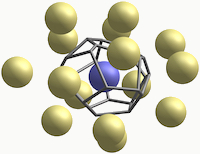### VoroTop Voronoi Cell Topology Visualization & Analysis Toolkit

##### Motivation

A common challenge in studying many physical systems is describing the "structure" of a set of points in space. Group theory provides powerful tools to study perfectly ordered systems, and probability theory provides tools to study "perfectly disordered" systems. Real systems, however, tend to be neither perfectly ordered not perfectly disordered. A meaningful way to describe this structure, in an elegant and practical manner, motivates the development of the Voronoi topology method.

##### Mathematical Background

Order parameters are commonly used to characterize local structure of individual points among other points in space. Each point is assigned a value, typically an integer, a real number, or set of several such numbers. Various order parameter values are then understood to indicate various types of local structure.

One example is centrosymmetry, which calculates how symmetric neighboring points are located relative to a central point. Bond-angle analysis, another order parameter commonly used, considers the angles formed between bonds of a central point. These approaches are typically useful at low temperatures, when the thermal vibrations are small when compared with structural defects. At temperatures above 20 to 40% of the melting temperature, however, deviations in the order parameter value that result from thermal flucations are of comparable magnitude to those resulting from structural defects, and so distinguishing thermal noise from structural abnormalities is not possible.

Moreover, conventional order parameters are typically most effective for highly ordered point sets. In such systems, most points have order-parameter values very close to some ideal order-parameter value. Points with order-parameter values that differ significantly from these ideal values can be treated as defects. However, disordered systems do not have ideal order-parameter values.

Complete information about the local neighborhood of a point can be kept by recording displacement vectors to its N closest neighbors, for some suitable choice of N. The complete structural information about a single point can itself be thought of as a point in a high-dimensional configuration space of dimension 3N. More specifically, we consider the configuration space: $$\mathcal C(n) = \{(\mathbf r_1, \mathbf r_2, ..., \mathbf r_n) : \mathbf r_i \in \mathbb{R}^3 \} /\operatorname{O}(3)/\Sigma_n,$$ where O(3) is the orthogonal group of three-dimensional rotations, and Σn is the symmetric group on n elements. These are here to ensure that arbitrary rotations of the neighboring points, and permutations of them, do not affect the description of structure. Scaling can also be mod'ed out.

Order parameters of the kind described above can be understood as mappings from this configuration space Pc to some order parameter space, often Rd or Zd, for some low d. Many order parameters, such as centrosymmetry and bond-angle analysis, are continuous functions on Pc. Although continuity of the order parameter provides certain nice properties (small perturbations in the structure result in small changes in the order parameter value), it also entails a very deep problem -- almost all points in the configuration space belong to level sets with arbitrarily large diameters. The upshot is that arbitrarily different structures will map to the same order parameter value.

## Voronoi cells

The Voronoi cell of a central particle is the region in space closer to that particle than to any other particle. Voronoi cells are used to study many physical systems.

## Voronoi cell topology

We use the term Voronoi cell topology to refer to its types of faces (triangules, rectangles, etc) and the way in which they are arranged.

## Weinberg vectors

In the 1960's, Louis Weinberg introduced an algorithm to efficiently test whether two planar graphs are isomorphic. This algorithm traces each graph and computes a "code" which captures complete topological data; two graphs are isomorphic if and only if their codes are identical. Since the vertex-edge graph of a Vornoi polyhedron is always planar, we use Weinberg's algorithm to completely encode the topology of each Voronoi cell; we call the codes which capture this information Weinberg vectors.

## Topological type

We say that two polyhedra have the same topological type if there is a one-to-one correspondance between their faces that perserves adjacency. For three-dimensional polyhedra, this is equivalent to saying that their edge-graphs are isomorphic.

## Filters

The set of topological types expected in a particular system can often be determined analytically. The Voronoi cells of many lattices, for example, are well known. Topological types of Voronoi cells of perturbed lattices can also be determined analytically. We use the word filter to refer to a list of topological types of a known structure, as we often use such a list to identify defects in otherwise ordered systems.